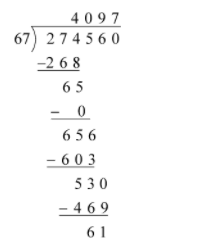QuestionAnswers

# The remainder obtained when $64 \times 65 \times 66$ is divide by 67 isA) 61B) 2C) 3D) 1

Hint: Here, we will first find the product of the given numbers and then divide the obtained product by the value mentioned in the question to find the required remainder from the division.

Complete step by step solution:
First, we will find the product of $64 \times 65 \times 66$.
$64 \times 65 \times 66 = 4160 \times 66 \\ = 274560 \\$
We know that the remainder is an amount, which is a left over after the division.

Dividing the above value by 67 to find the remainder, we getThus, we get that the remainder of this division is 61.

Hence, the option A is correct.

Note: In this question, we have to calculate the product first then divide the obtained solution by the given number. Some students find the division before the multiplication, which is wrong.